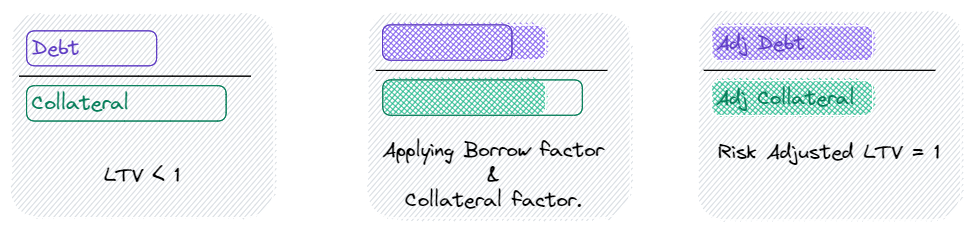Comment on page

# Loan to Value (LTV)

Notional is an overcollateralized lending protocol. This means that the maximum amount you can borrow is less than the total value of your collateral. Your Loan to Value (LTV) is the value of your loan in USD divided by the value of your collateral in USD.
$LTV = loanValue / collateralValue$
For example, if you borrowed 100 USDC against 200 USDC worth of ETH, your LTV would be 50%.
// Example LTV calculation
loan value = 100 USDC
collateral value = 200 USDC
LTV = 100 / 200 = 0.5

### Max LTV

The maximum amount you can borrow against your collateral is called the Max LTV. If the Max LTV was 75%, you could borrow another 50 USDC before you would reach your max borrow capacity.
// User at Max LTV of 75%
loan value = 150 USDC
collateral value = 200 USDC
LTV = 150 / 200 = 0.75
Your Max LTV depends on what assets you're using as collateral, what you're borrowing, and Notional's risk parameters.

### Calculating Max LTV

To calculate the risk-adjusted LTV, Notional applies a collateral factor to your collateral value and a borrow factor to your debt value that depend on what asset you're using as collateral and what asset you're borrowing.
$riskAdjustedLTV = loanValue*borrowFactor / collateralValue*collateralFactor$
All borrow factors are greater than 1, and all collateral factors are less than 1. Applying these factors increases the value of your debt, decreases the value of your collateral, and makes the risk-adjusted LTV greater than your regular LTV.

### Max LTV calculation example

Here's an example of an account whose LTV is less than 1 but risk-adjusted LTV equals 1:In this example, the account is at its max LTV because it's risk-adjusted LTV equals 1.
Here is an example of how you would calculate Max LTV numerically.
// Max LTV calculation example
loan value = 100 USDC
collateral value = 200 USDC
borrow factor = 1.1
collateral factor = 0.825
LTV = 100 / 200 = 0.5
Risk-adjusted LTV = 100 * 1.1 / 200 * 0.8 = 110 / 165 = 0.667
Max LTV = 0.5 / 0.667 = 0.75